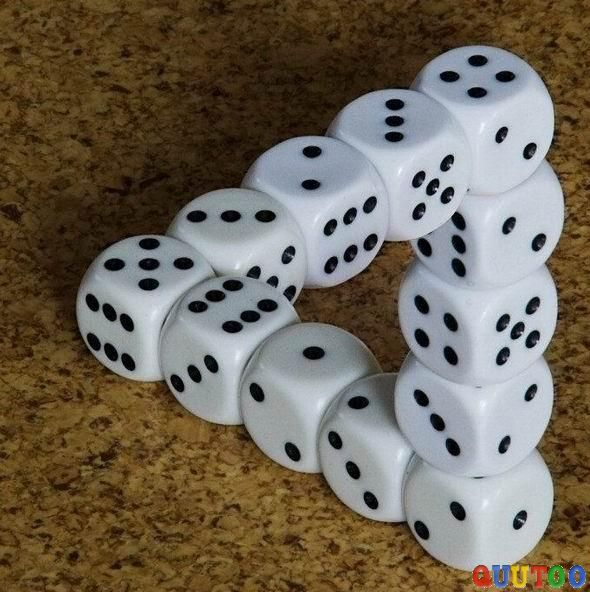# Dice Numbers Creating Triangles

Probability Level 5Potsawee has a fair six-sided die. He throws the die 3 times, and the numbers shown on the upper side each time are $a,b,c$ respectively. Find the probability that the lengths $(a,b,c)$ are able to create an isosceles triangle. Give your answer to three significant figures.

×